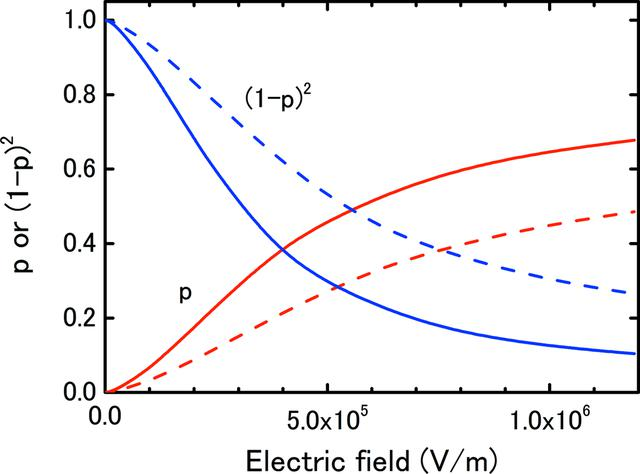disable zoom     view article Figure 8 Free-electron fraction, p, and (1 − p)2 as a function of the electric field calculated using equations (14)and (15). The solid and broken lines indicate the results for d = 4.2 and 8.4 mm. The factor of (1 − p)2 is the ratio of K in equation (6)to that in equation (13), i.e. the rate of change of K.JOURNAL OFSYNCHROTRONRADIATION
ISSN: 1600-5775
Volume 20| Part 5| July 2013| Pages 698-704